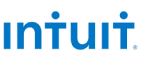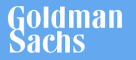New update is available. Click here to update.

# Balanced parentheses

Contributed by
Deepanshu_1780
Medium0/80
10 mins
90 %11 upvotes+54 more

## Problem Statement

#### Note :

``````Conditions for valid parentheses:
1. All open brackets must be closed by the closing brackets.

2. Open brackets must be closed in the correct order.
``````

#### For Example :

``````()()()() is a valid parentheses.
)()()( is not a valid parentheses.
``````
Detailed explanation ( Input/output format, Notes, Images )
##### Constraints:
``````1 <= T <= 5
1 <= N <= 10
Time Limit : 1 sec.
``````
##### Sample Input 1 :
``````2
2
3
``````
##### Sample Output 1 :
``````(())
()()
((()))
(()())
(())()
()(())
()()()
``````
##### Explanation for Sample Input 1 :
``````Test Case 1:

For 2 pair of parentheses the combinations of balanced parentheses will be (()) ()()

Test Case 2:

For 3 pair of parentheses the combinations of balanced parentheses will be
((())) (()()) (())() ()(()) ()()()
``````
##### Sample Input 2 :
``````2
1
4
``````
##### Sample Output 2 :
``````()
(((())))
((()()))
((())())
((()))()
(()(()))
(()()())
(()())()
(())(())
(())()()
()((()))
()(()())
()(())()
()()(())
()()()()
``````AutocompleteConsole